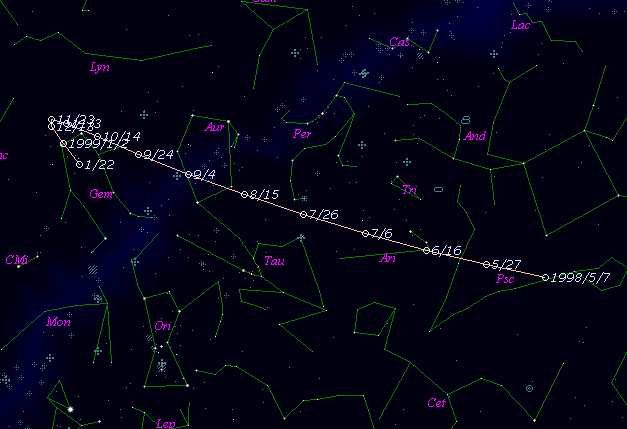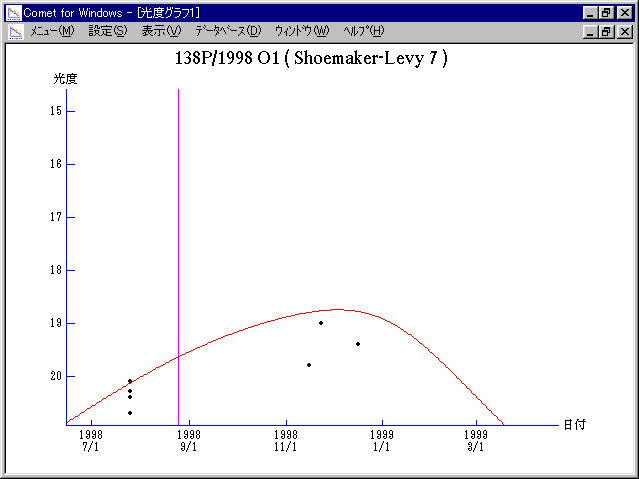# \$B%7%e!<%a!<%+!<(B-\$B%l%t%#Bh#7WB@1(B

138P/Shoemaker-Levy 7 (1998)###\$B%W%m%U%#!<%k(B

 \$BId9f(B 138P/1998 O1 \$B8!=PF|(B 1998\$BG/(B 7\$B7n(B25\$BF|(B \$B8!=P8wEY(B 20.7\$BEy(B \$B8!=P J. V. Scotti (Lunar and Planetary Laboratory)

###\$B50F;MWAG(B

```   The following improved orbital elements by Kenji Muraoka, are
from 44 observations 1991 Nov. 13 to 1998 Dec. 16, perturbations
by 9 Plantes, Moon and 5 minor planets were taken into account.
The mean residual is +/- 0.69 arc seconds.

Epoch  =  1998 Aug. 15.0  TT       JDT = 2451040.5
T  =  1998 Aug. 24.56639       +/- 0.00125 (m.e.) TT
Peri. =   95.52316                +/- 0.00072
Node  =  309.51785                +/- 0.00035   (2000.0)
Incl. =   10.08888                +/- 0.00004
q  =    1.6971999              +/- 0.0000039 AU
e  =    0.5311206              +/- 0.0000012
a  =    3.6196936              +/- 0.0000034 AU
n  =    0.14311869             +/- 0.00000020
P  =    6.887                  +/- 0.0000098  years
```

###\$B@1?^(B###\$B8wEYJQ2=(B

```        m1 = 16.0 + 5 log\$B&\$(B + 10.0 log r(t - 50)
```##### \$B50F;MWAG\$OB<2,7r<#;a\$N7W;;\$K\$h\$k\$b\$N\$G\$9!#(B \$B@1?^\$O(B StellaNavigator Ver.2.0 for Windows (\$B%"%9%H%m%"!<%D(B \$BJTCx(B / \$B%"%9%-!<=PHG6I4)(B) \$B\$G:n@.\$7\$?\$b\$N\$G\$9!#(B \$B8wEY%0%i%U\$O(BComet for Windows\$B\$G:n@.\$7\$?\$b\$N\$G\$9!#(B